400万+工程师在用400万+工程师在用

# 算法工程师须知的十个炼丹trick33人已加入

### 描述

Focal Loss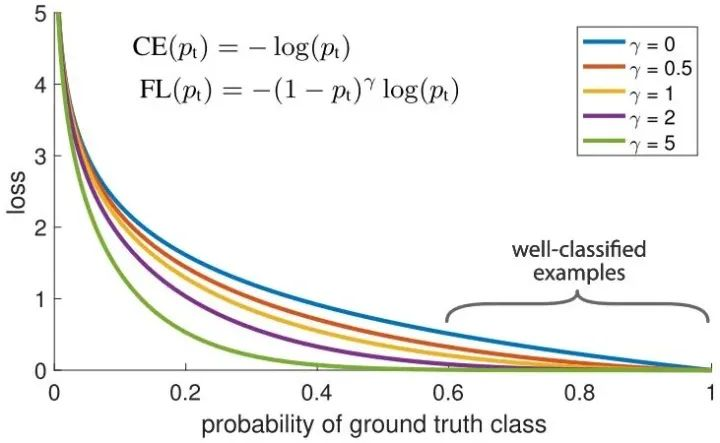loss = -np.log(p) loss = (1-p)^G * loss

Dropout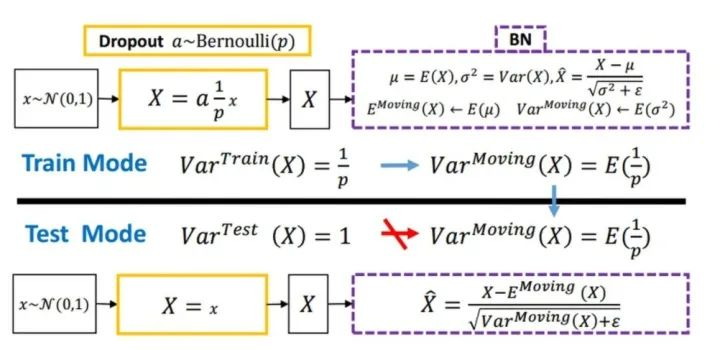Normalization

Batch Normalization 于2015年由 Google 提出，开 Normalization 之先河。其规范化针对单个神经元进行，利用网络训练时一个 mini-batch 的数据来计算该神经元的均值和方差,因而称为 Batch Normalization。

x = (x - x.mean()) / x.std()

relu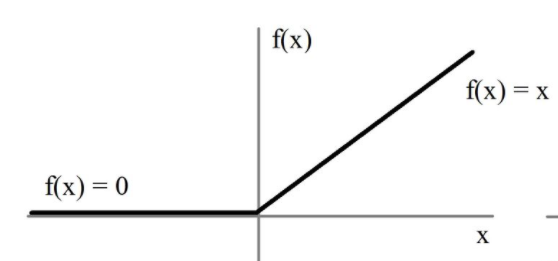x = max(x, 0)

Cyclic LR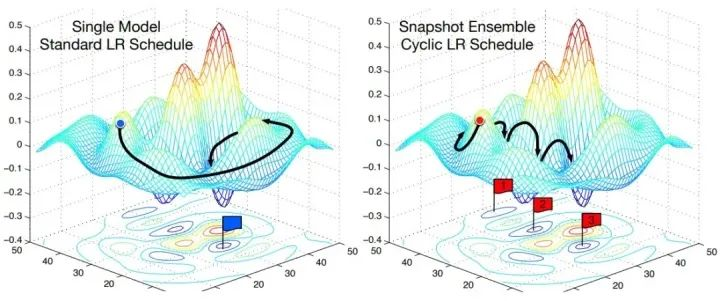scheduler = lambda x: ((LR_INIT-LR_MIN)/2)*(np.cos(PI*(np.mod(x-1,CYCLE)/(CYCLE)))+1)+LR_MIN

With Flooding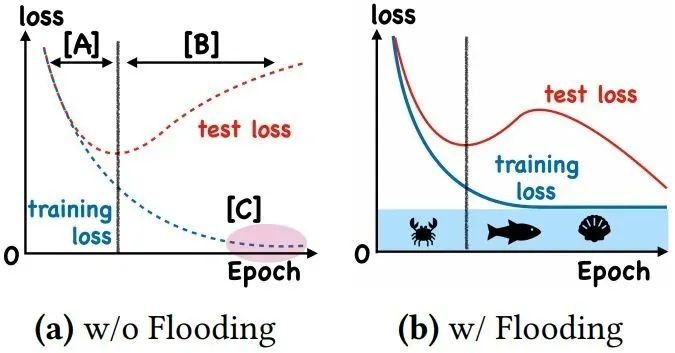flood = (loss - b).abs() + b

Group Normalization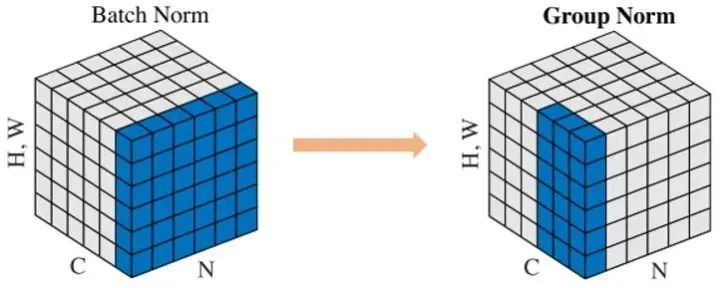Face book AI research（FAIR）吴育昕-恺明联合推出重磅新作Group Normalization（GN），提出使用Group Normalization 替代深度学习里程碑式的工作Batch normalization。一句话概括，Group Normbalization（GN）是一种新的深度学习归一化方式，可以替代BN。

def GroupNorm(x, gamma, beta, G, eps=1e-5): # x: input features with shape [N,C,H,W] # gamma, beta: scale and offset, with shape [1,C,1,1] # G: number of groups for GN N, C, H, W = x.shape x = tf.reshape(x, [N, G, C // G, H, W]) mean, var = tf.nn.moments(x, [2, 3, 4], keep dims=True) x = (x - mean) / tf.sqrt(var + eps) x = tf.reshape(x, [N, C, H, W])    return x * gamma + beta

Label Smoothing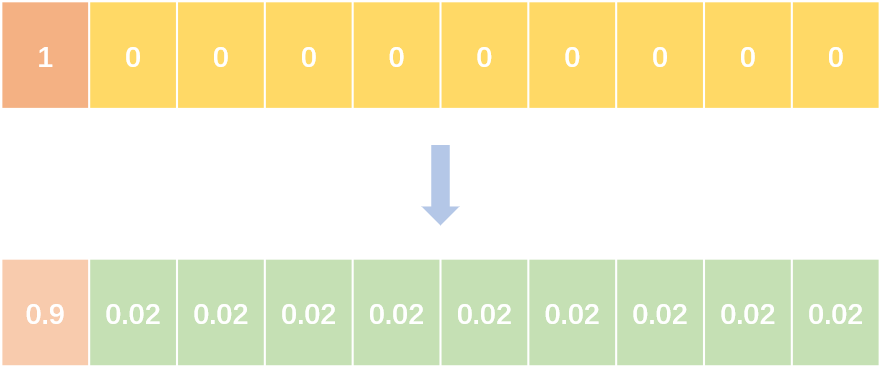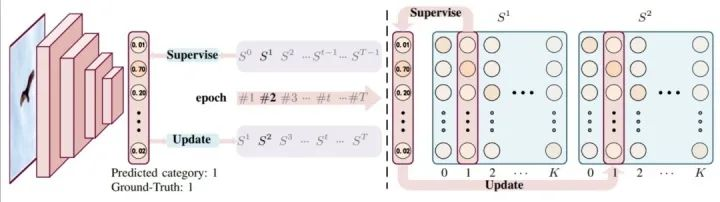abel smoothing将hard label转变成soft label，使网络优化更加平滑。标签平滑是用于深度神经网络（DNN）的有效正则化工具，该工具通过在均匀分布和hard标签之间应用加权平均值来生成soft标签。它通常用于减少训练DNN的过拟合问题并进一步提高分类性能。

targets = (1 - label_smooth) * targets + label_smooth / num_classes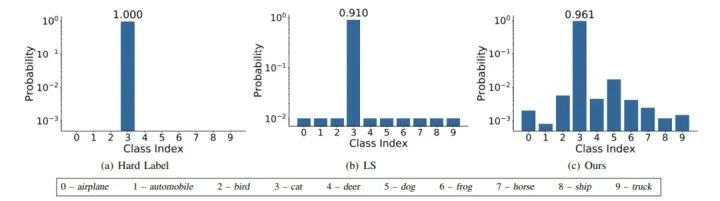Wasserstein GAN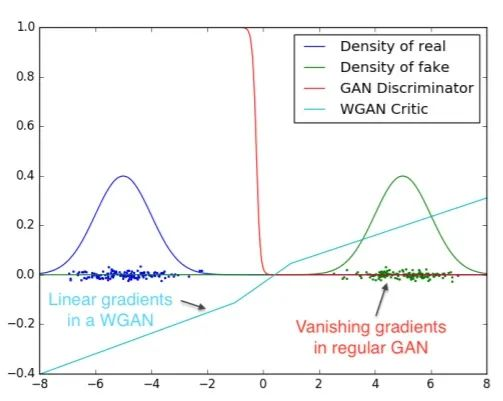Skip Connection

F(x) = F(x) + x